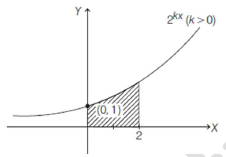If the area above the X-axis bounded by the curves y=2kx,x=0 and x=2 is 3log 2  then the value of k is

# If the area above the X-axis bounded by the curves   then the value of k is

1. A

$\frac{1}{2}$

2. B

-1

3. C

1

4. D

2

Fill Out the Form for Expert Academic Guidance!l

+91

Live ClassesBooksTest SeriesSelf Learning

Verify OTP Code (required)

### Solution:+91

Live ClassesBooksTest SeriesSelf Learning

Verify OTP Code (required)